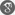##### Cite as:
Bapurao C. Dhage, Dhage Iteration Method for Generalized Quadratic Functional Integral Equations, Int. J. Anal. Appl., 7 (1) (2015), 59-69.

#### Abstract

In this paper we prove the existence as well as approximations of the solutions for a certain nonlinear generalized quadratic functional integral equation. An algorithm for the solutions is developed and it is shown that the sequence of successive approximations starting at a lower or upper solution converges monotonically to the solutions of related quadratic functional integral equation under some suitable mixed hybrid conditions. We rely our main result on Dhage iteration method embodied in a recent hybrid fixed point theorem of Dhage (2014) in partially ordered normed linear spaces. An example is also provided to illustrate the abstract theory developed in the paper.

#### References

1. I. K. Argyros, Quadratic equations and applications to Chandrasekhar’s and related equations, Bull. Austral. Math. Soc. 32 (1985), 275-292.2. S. Chandrasekher, Radiative Transfer, Dover Publications, New York, 1960.3. K. Deimling, Nonlinear Fuctional Analysis, Springer-Verlag, Berlin, 1985.4. B.C. Dhage, On α-condensing mappings in Banach algebras, The Mathematics Student 63 (1994), 146-152.5. B.C. Dhage, Fixed point theorems in ordered Banach algebras and applications, PanAmer. Math. J. 9(4) (1999), 93-102.6. B.C. Dhage, A fixed point theorem in Banach algebras with applications to functional integral equations, Kyungpook Math. J. 44 (2004), 145-155.7. B.C. Dhage, A nonlinear alternative in Banach algebras with applications to functional differential equations, Nonlinear Funct. Anal. & Appl. 8 (2004), 563–575.8. B. C. Dhage, Periodic boundary value problems of first order Carath´eodory and discontinuous differential equations, Nonlinear Funct. Anal. & Appl. 13(2) (2008), 323-352.9. B.C. Dhage, Hybrid fixed point theory in partially ordered normed linear spaces and applications to fractional integral equations, Differ. Equ Appl. 5 (2013), 155-184.10. B.C. Dhage, Partially condensing mappings in ordered normed linear spaces and applications to functional integral equations, Tamkang J. Math. 45 (4) (2014), 397-426.11. B.C. Dhage, Nonlinear D-set-contraction mappings in partially ordered normed linear spaces and applications to functional hybrid integral equations, Malaya J. Mat. 3(1)(2015), 62-85.12. B.C. Dhage, Operator theoretic techniques in the theory of nonlinear hybrid differential equations, Nonlinear Anal. Forum 20 (2015), 15-31.13. S. Heikkil¨a, V. Lakshmikantham, Monotone Iterative Techniques for Discontinuous Nonlinear Differential Equations, Marcel Dekker inc., New York 1994.